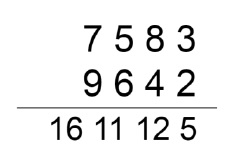# Addition of two numbers without propagating Carry?

CServer Side ProgrammingProgramming

#### C in Depth: The Complete C Programming Guide for Beginners

45 Lectures 4.5 hours

#### Practical C++: Learn C++ Basics Step by Step

Most Popular

50 Lectures 4.5 hours

#### Master C and Embedded C Programming- Learn as you go

66 Lectures 5.5 hours

Here we will see one problem, where we will add two n digit numbers but the carry will not be propagated. We can understand this concept through an example −So we can see that here only digits are getting added and answer is placed. Here is one trick. We have to scan the numbers from right to left. So the sum of 3+2 = 6 will be calculated first, but it will be placed at the end. So we will use stack to store intermediate results.

## Algorithm

#### noPropagateCarry(a, b)

begin
size = max of length of a and length of b
for i in range i to size, do
al := last digit of a
bl := last digit of b
push (al + bl) into stack
a := a / 10
b := b /10
done
pop and print the elements from stack
end

## Example

Live Demo

#include<iostream>
#include<stack>
#include<cmath>
using namespace std;
int length(int n){
return log10(n) + 1;
}
void noPropagateCarry(int a, int b){
int size = max(length(a), length(b));
stack<int> stk;
for(int i = 0; i <size; i++){
int al = a % 10; //last digit of a
int bl = b % 10; //last digit of b
stk.push(al + bl);
a = a / 10; b = b/10;
}
while(!stk.empty()){
cout << stk.top();
stk.pop();
}
}
main() {
int a = 7583, b = 9642;
cout << "Result: ";
noPropagateCarry(a, b);
}

## Output

Result: 1611125